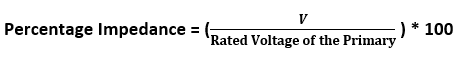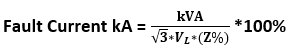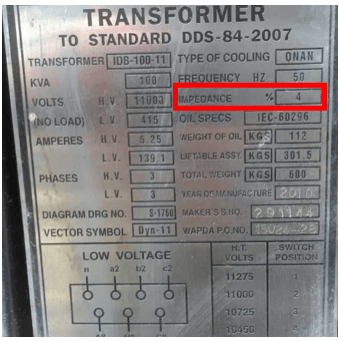# What is transformer impedance?

A transformer’s primary and secondary windings have resistance and reactance, collectively referred to as impedance. The impedance opposes the flow of current when the transformer is connected to an AC power supply, causing a voltage drop across the windings.

When an AC voltage is applied to the primary winding, it generates a magnetic flux. However, not all of this flux is fully linked to both windings; some amount of flux leaks. This leakage flux is represented by the leakage reactance in the transformer. Consequently, the transformer windings possess both resistance and reactance, contributing to the overall impedance.

During operation, the impedance of the transformer opposes the current flow, resulting in a voltage drop in the windings. The magnitude of this voltage drop is directly related to the impedance or percentage impedance of the transformer. The percentage impedance signifies the percentage of the rated voltage required to circulate the rated current through one winding when the other winding is short-circuited.

## How to measure the transformer impedance practically?

The percentage impedance of a transformer is typically determined by conducting a short circuit test. However, it is important to note that applying the full rated voltage to the primary side during this test can lead to severe damage to the transformer due to the excessive short circuit current.

To calculate the percentage impedance safely, a different setup is used. The secondary winding is short-circuited, and the voltage is gradually increased on the high-voltage (HV) side of the transformer. The primary voltage should be increased incrementally from zero volts until the current in the secondary winding reaches the full load rated current.

At this point, the primary voltage (V) is noted down when the secondary current reaches the transformer’s full load current. This primary voltage is typically much lower than the full rated voltage to avoid any damage to the transformer during the test.

The percentage impedance of the transformer can be calculated using the following formula:Where:

• V is the primary voltage at which the secondary current reaches the full load current
• Rated Voltage is the full rated voltage of the transformer primary

By applying this formula, the percentage impedance of the transformer can be determined accurately and safely, providing valuable information about the transformer’s electrical characteristics and performance.

To illustrate this, let’s consider a transformer with a primary winding rated at 11000 Volts. When this transformer is fully loaded, a measured primary voltage of 450 Volts is observed. The percentage voltage drop (Impedance) can be calculated as,Hence, we can conclude that the transformer’s percentage impedance is 4%.

## Percentage Impedance for Short Circuit Calculation

The percentage impedance of a transformer plays a significant role in short circuit calculations by limiting the fault current magnitude. A higher percentage impedance results in a lower fault current magnitude, while a lower percentage impedance allows for a higher fault current.

## Transformer Fault Current:

The fault current at the secondary side of the transformer can be expressed using the following mathematical relationship:Where:

Fault kVA represents the fault level at the secondary side of the transformer in kilovolt-amperes (kVAsc)

Percentage Impedance refers to the percentage impedance of the transformer (Z %)

For instance, consider an 11kV/ kV, 100 kVA transformer with a percentage impedance of 4%. By utilizing the above formula, the fault level at the secondary side of the transformer can be calculated.Transformer fault level calculation makes an assumption that the transformer is supplied from an infinite bus where the fault level on the primary side of the transformer is considered to be infinite. By adopting this assumption, the calculator provides a worst-case fault level on the secondary side of the transformer. This worst-case value is sufficient for the sizing of equipment based on fault ratings, ensuring adequate protection in the system.

## Further classification of fault current calculationThe percentage impedance (%Z) of a transformer holds significant importance in various aspects of its operation and protection:

• Limiting Short Circuit Current: A higher percentage impedance leads to a reduced short circuit current magnitude. This characteristic helps protect the system and connected equipment by restricting the flow of excessive fault currents.
• Voltage Drop and Voltage Regulation: A higher percentage impedance causes more voltage drop within the transformer. This increased voltage drop can result in poorer voltage regulation, meaning that the transformer may struggle to maintain the desired voltage levels under varying load conditions. Consequently, higher %Z values can lead to decreased efficiency and potential issues with equipment operation.
• Protection Design: The %Z is a crucial parameter utilized in the design of protection switchgear. By considering the %Z, the maximum level of fault current can be calculated. This information aids in determining the appropriate specifications and capabilities of the switchgear required to safely withstand and interrupt the maximum fault current levels.
Scroll to Top